# myppt-120101023904-phpapp01.pdf

22 Nov 2022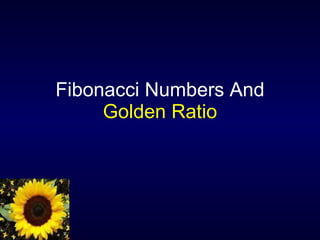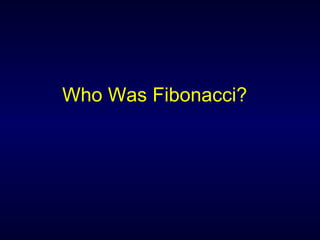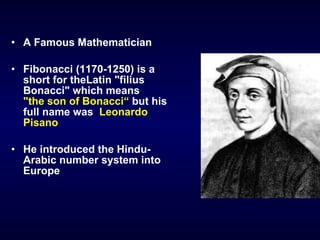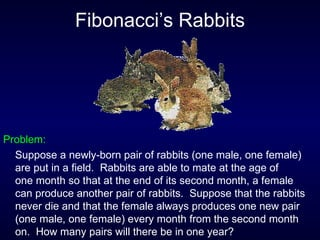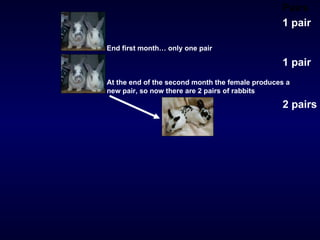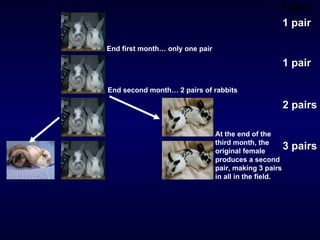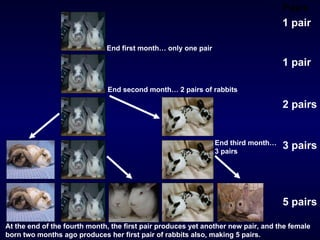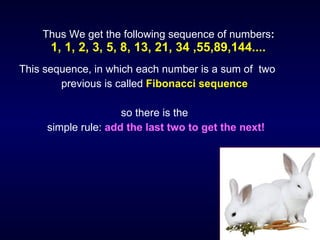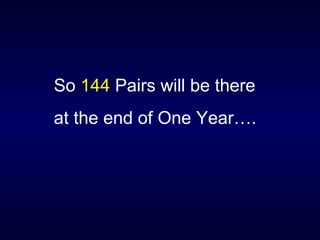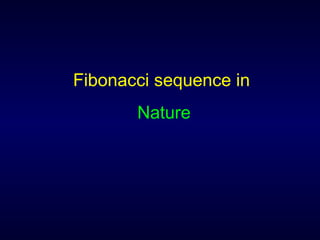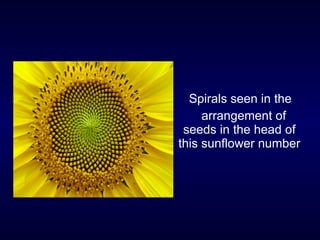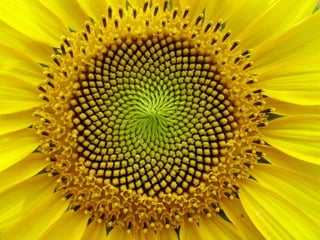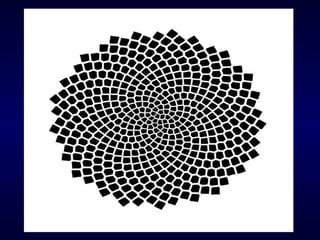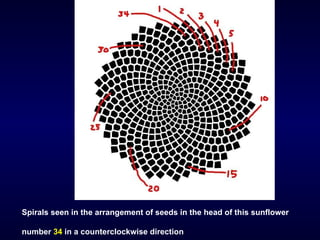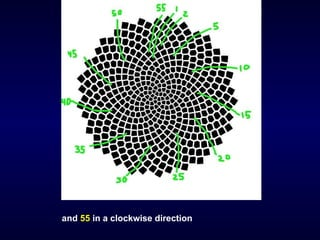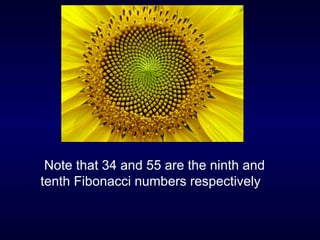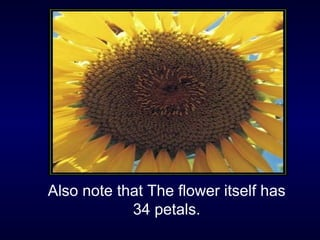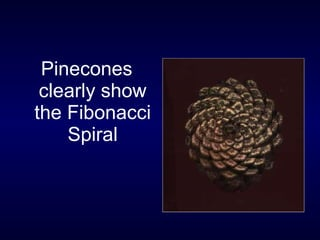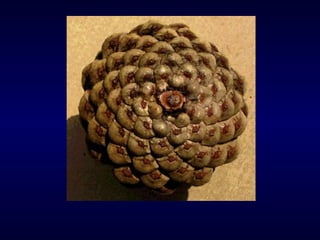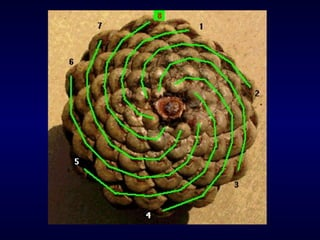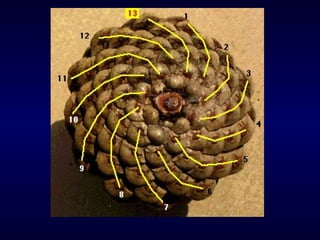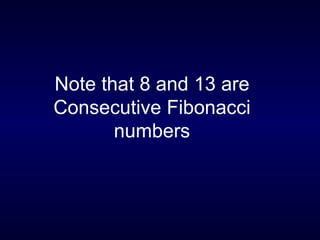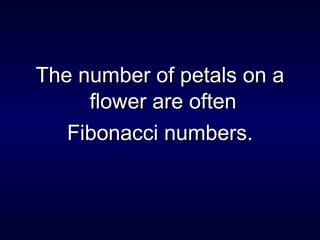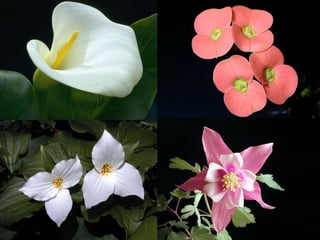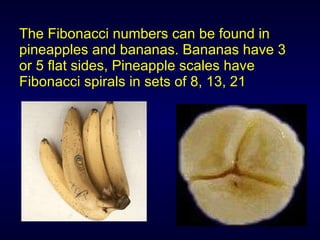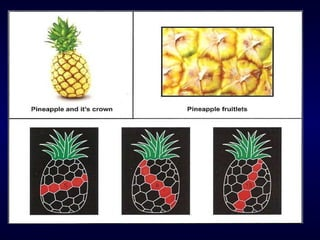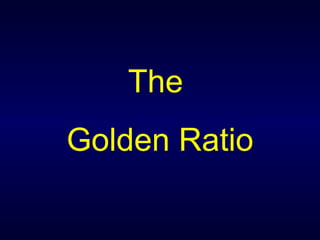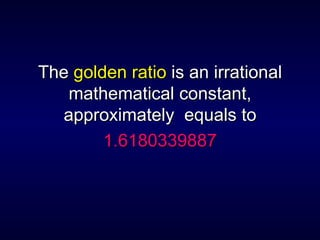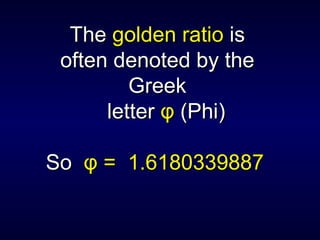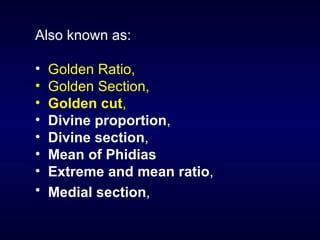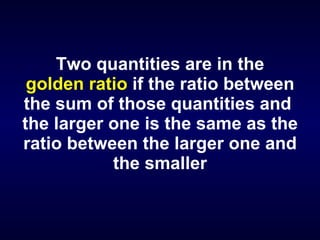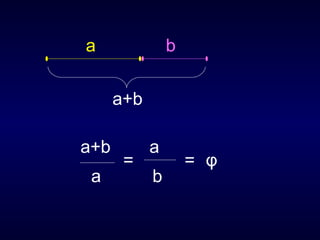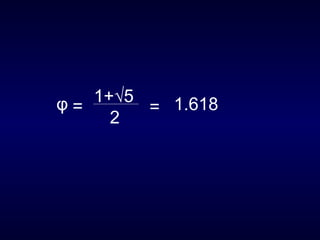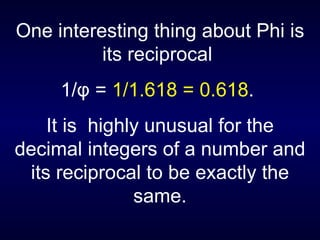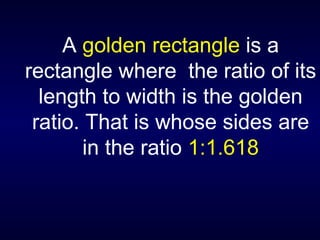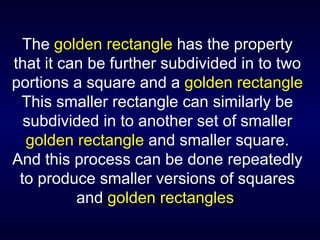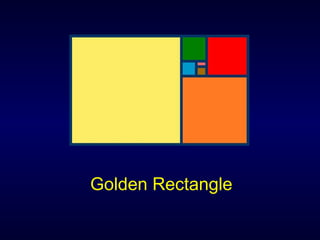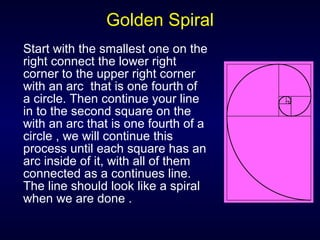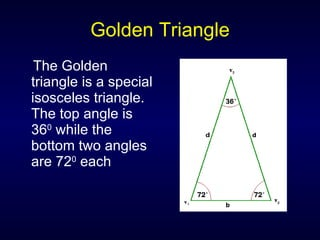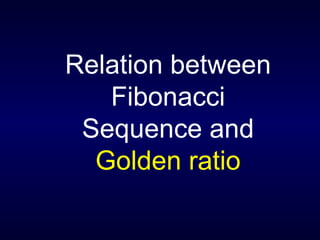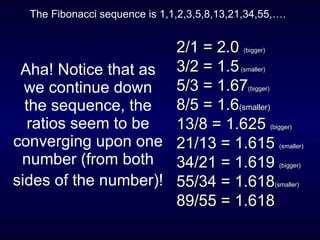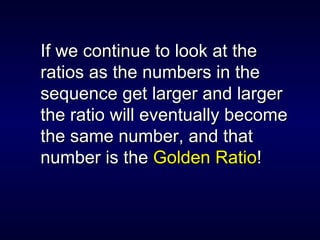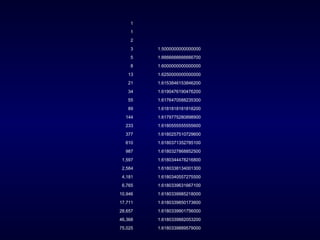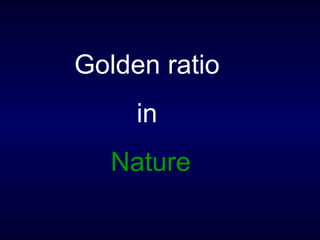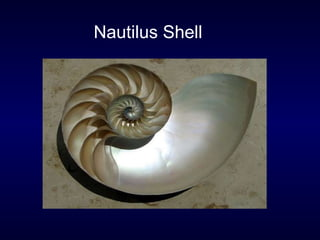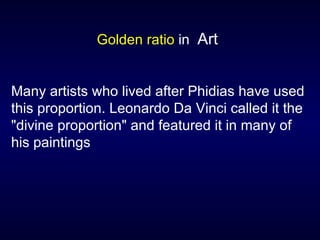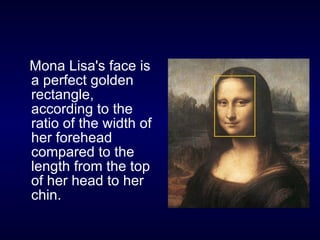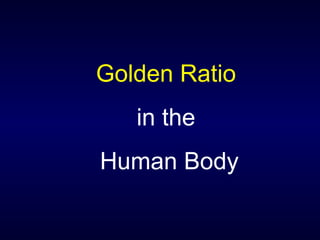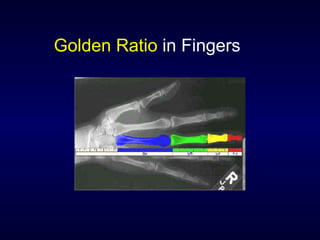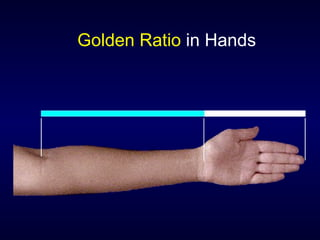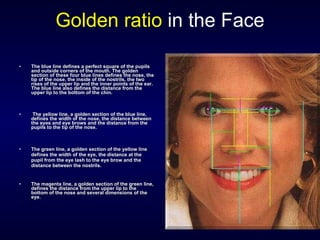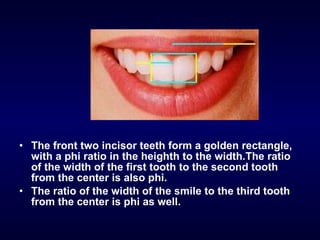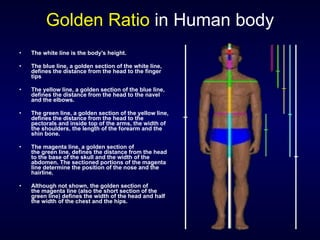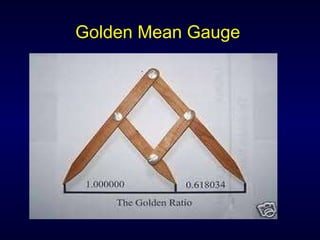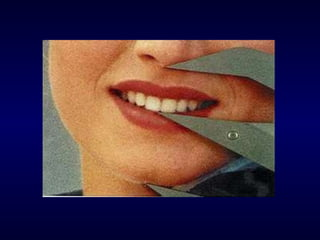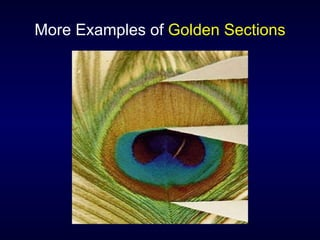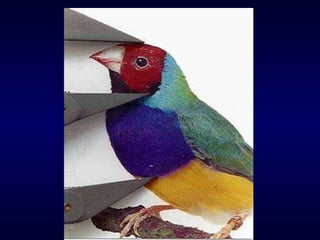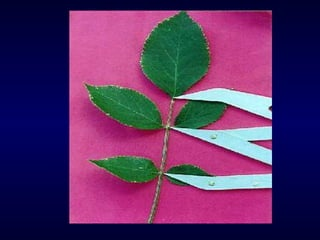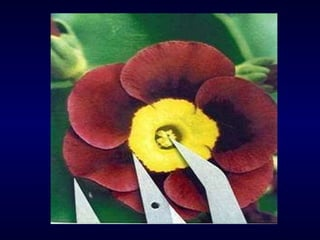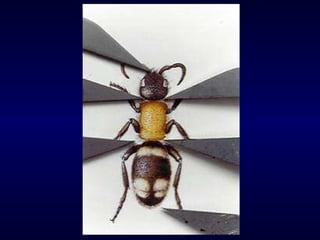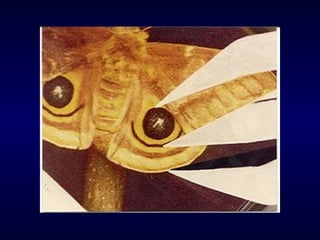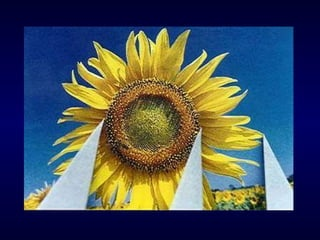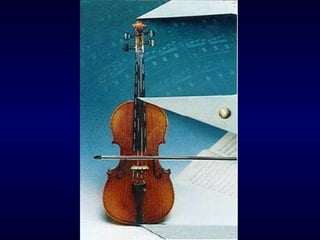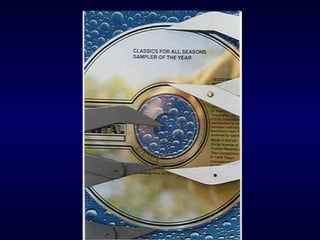1 sur 71

### myppt-120101023904-phpapp01.pdf

• 4. • A Famous Mathematician • Fibonacci (1170-1250) is a short for theLatin "filius Bonacci" which means "the son of Bonacci“ but his full name was Leonardo Pisano • He introduced the Hindu- Arabic number system into Europe
• 6. Fibonacci Sequence was discovered after an investigation on the reproduction of rabbits.
• 7. Fibonacci’s Rabbits Problem: Suppose a newly-born pair of rabbits (one male, one female) are put in a field. Rabbits are able to mate at the age of one month so that at the end of its second month, a female can produce another pair of rabbits. Suppose that the rabbits never die and that the female always produces one new pair (one male, one female) every month from the second month on. How many pairs will there be in one year?
• 8. Pairs 1 pair At the end of the first month there is still only one pair
• 9. Pairs 1 pair 1 pair 2 pairs End first month… only one pair At the end of the second month the female produces a new pair, so now there are 2 pairs of rabbits
• 10. Pairs 1 pair 1 pair 2 pairs 3 pairs End second month… 2 pairs of rabbits At the end of the third month, the original female produces a second pair, making 3 pairs in all in the field. End first month… only one pair
• 11. Pairs 1 pair 1 pair 2 pairs 3 pairs End third month… 3 pairs 5 pairs End first month… only one pair End second month… 2 pairs of rabbits At the end of the fourth month, the first pair produces yet another new pair, and the female born two months ago produces her first pair of rabbits also, making 5 pairs.
• 12. Thus We get the following sequence of numbers: 1, 1, 2, 3, 5, 8, 13, 21, 34 ,55,89,144.... This sequence, in which each number is a sum of two previous is called Fibonacci sequence so there is the simple rule: add the last two to get the next!
• 13. So 144 Pairs will be there at the end of One Year….
• 15. Spirals seen in the arrangement of seeds in the head of this sunflower number
• 18. Spirals seen in the arrangement of seeds in the head of this sunflower number 34 in a counterclockwise direction
• 19. and 55 in a clockwise direction
• 20. Note that 34 and 55 are the ninth and tenth Fibonacci numbers respectively.
• 21. Also note that The flower itself has 34 petals.
• 26. Note that 8 and 13 are Consecutive Fibonacci numbers
• 27. The number of petals on a The number of petals on a flower are often flower are often Fibonacci numbers. Fibonacci numbers.
• 30. The Fibonacci numbers can be found in pineapples and bananas. Bananas have 3 or 5 flat sides, Pineapple scales have Fibonacci spirals in sets of 8, 13, 21
• 33. The The golden ratio golden ratio is an irrational is an irrational mathematical constant, mathematical constant, approximately equals to approximately equals to 1.6180339887 1.6180339887
• 34. The The golden ratio golden ratio is is often denoted by the often denoted by the Greek Greek letter letter φ φ (Phi) (Phi) So So φ = φ = 1.6180339887 1.6180339887
• 35. Also known as: • Golden Ratio, • Golden Section, • Golden cut, • Divine proportion, • Divine section, • Mean of Phidias • Extreme and mean ratio, • Medial section,
• 36. Two quantities are in the golden ratio if the ratio between the sum of those quantities and the larger one is the same as the ratio between the larger one and the smaller .
• 39. One interesting thing about Phi is its reciprocal 1/φ = 1/1.618 = 0.618. It is highly unusual for the decimal integers of a number and its reciprocal to be exactly the same.
• 40. A golden rectangle is a rectangle where the ratio of its length to width is the golden ratio. That is whose sides are in the ratio 1:1.618
• 41. The golden rectangle has the property that it can be further subdivided in to two portions a square and a golden rectangle This smaller rectangle can similarly be subdivided in to another set of smaller golden rectangle and smaller square. And this process can be done repeatedly to produce smaller versions of squares and golden rectangles
• 43. Golden Spiral Start with the smallest one on the right connect the lower right corner to the upper right corner with an arc that is one fourth of a circle. Then continue your line in to the second square on the with an arc that is one fourth of a circle , we will continue this process until each square has an arc inside of it, with all of them connected as a continues line. The line should look like a spiral when we are done .
• 44. Golden Triangle The Golden triangle is a special isosceles triangle. The top angle is 360 while the bottom two angles are 720 each
• 46. Aha! Notice that as we continue down the sequence, the ratios seem to be converging upon one number (from both sides of the number)! 2/1 = 2.0 2/1 = 2.0 (bigger) (bigger) 3/2 = 1.5 3/2 = 1.5(smaller) (smaller) 5/3 = 1.67 5/3 = 1.67(bigger) (bigger) 8/5 = 1.6 8/5 = 1.6(smaller) (smaller) 13/8 = 1.625 13/8 = 1.625 (bigger) (bigger) 21/13 = 1.615 21/13 = 1.615 (smaller) (smaller) 34/21 = 1.619 34/21 = 1.619 (bigger) (bigger) 55/34 = 1.618 55/34 = 1.618(smaller) (smaller) 89/55 = 1.618 89/55 = 1.618 The Fibonacci sequence is 1,1,2,3,5,8,13,21,34,55,….
• 47. If we continue to look at the If we continue to look at the ratios as the numbers in the ratios as the numbers in the sequence get larger and larger sequence get larger and larger the ratio will eventually become the ratio will eventually become the same number, and that the same number, and that number is the number is the Golden Ratio Golden Ratio! !
• 48. 1 1 2 3 1.5000000000000000 5 1.6666666666666700 8 1.6000000000000000 13 1.6250000000000000 21 1.6153846153846200 34 1.6190476190476200 55 1.6176470588235300 89 1.6181818181818200 144 1.6179775280898900 233 1.6180555555555600 377 1.6180257510729600 610 1.6180371352785100 987 1.6180327868852500 1,597 1.6180344478216800 2,584 1.6180338134001300 4,181 1.6180340557275500 6,765 1.6180339631667100 10,946 1.6180339985218000 17,711 1.6180339850173600 28,657 1.6180339901756000 46,368 1.6180339882053200 75,025 1.6180339889579000
• 51. Golden ratio in Art Many artists who lived after Phidias have used this proportion. Leonardo Da Vinci called it the "divine proportion" and featured it in many of his paintings
• 52. Mona Lisa's face is a perfect golden rectangle, according to the ratio of the width of her forehead compared to the length from the top of her head to her chin.
• 54. Golden Ratio in Fingers
• 56. Golden ratio in the Face • The blue line defines a perfect square of the pupils The blue line defines a perfect square of the pupils and outside corners of the mouth. The golden and outside corners of the mouth. The golden section of these four blue lines defines the nose, the section of these four blue lines defines the nose, the tip of the nose, the inside of the nostrils, the two tip of the nose, the inside of the nostrils, the two rises of the upper lip and the inner points of the ear. rises of the upper lip and the inner points of the ear. The blue line also defines the distance from the The blue line also defines the distance from the upper lip to the bottom of the chin. upper lip to the bottom of the chin. • The yellow line, a golden section of the blue line, The yellow line, a golden section of the blue line, defines the width of the nose, the distance between defines the width of the nose, the distance between the eyes and eye brows and the distance from the the eyes and eye brows and the distance from the pupils to the tip of the nose. pupils to the tip of the nose. • The green line, a golden section of the yellow line The green line, a golden section of the yellow line defines the width of the eye, the distance at the defines the width of the eye, the distance at the pupil from the eye lash to the eye brow and the pupil from the eye lash to the eye brow and the distance between the nostrils. distance between the nostrils. • The magenta line, a golden section of the green line, The magenta line, a golden section of the green line, defines the distance from the upper lip to the defines the distance from the upper lip to the bottom of the nose and several dimensions of the bottom of the nose and several dimensions of the eye. eye.
• 57. • The front two incisor teeth form a golden rectangle, with a phi ratio in the heighth to the width.The ratio of the width of the first tooth to the second tooth from the center is also phi. • The ratio of the width of the smile to the third tooth from the center is phi as well.
• 58. Golden Ratio in Human body • The white line is the body's height. • The blue line, a golden section of the white line, defines the distance from the head to the finger tips • The yellow line, a golden section of the blue line, defines the distance from the head to the navel and the elbows. • The green line, a golden section of the yellow line, defines the distance from the head to the pectorals and inside top of the arms, the width of the shoulders, the length of the forearm and the shin bone. • The magenta line, a golden section of the green line, defines the distance from the head to the base of the skull and the width of the abdomen. The sectioned portions of the magenta line determine the position of the nose and the hairline. • Although not shown, the golden section of the magenta line (also the short section of the green line) defines the width of the head and half the width of the chest and the hips.
• 61. More Examples of Golden Sections